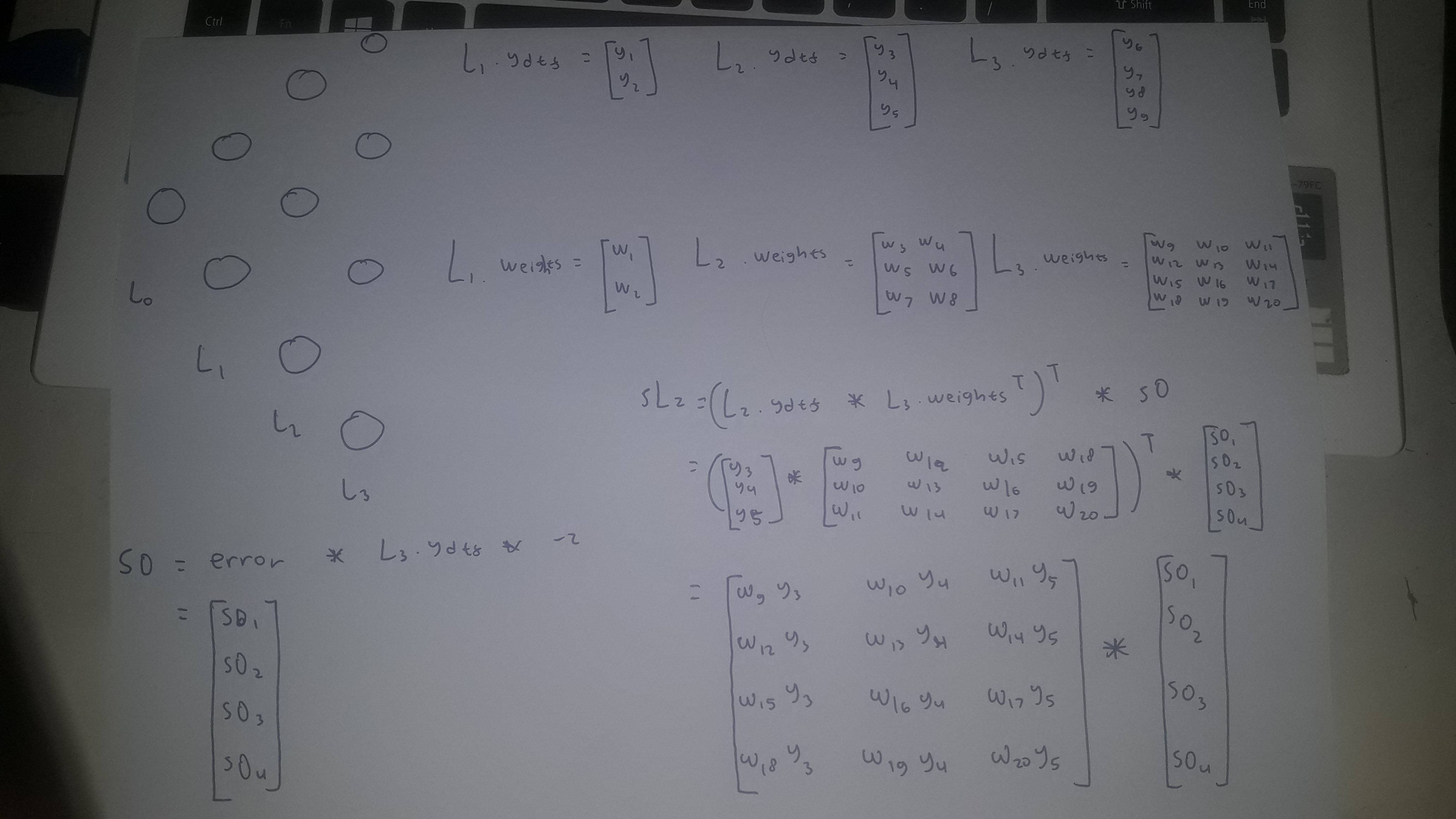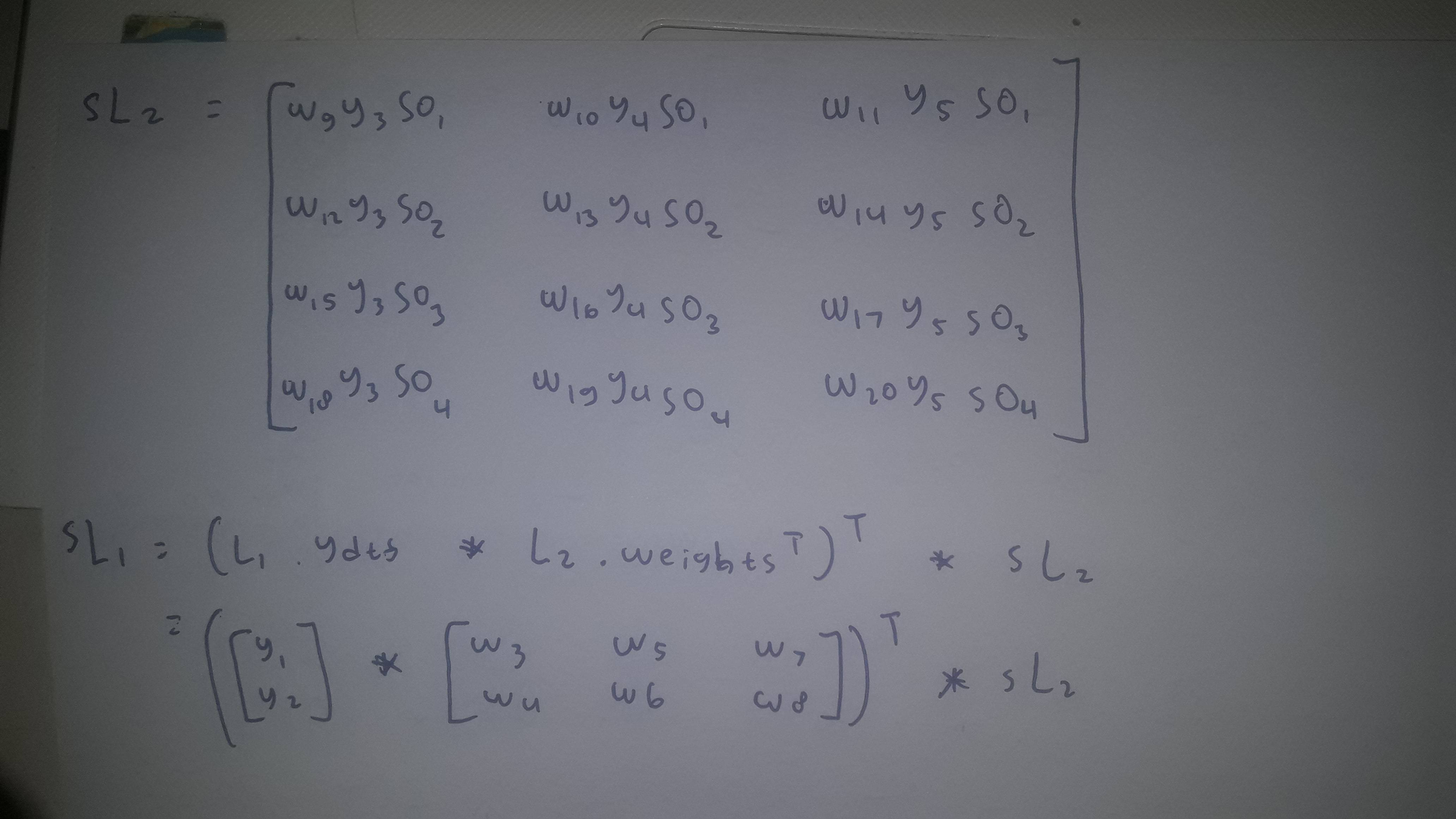# How does multiplying matrices in backpropagation work

I'm new to neural networks and im tasked to create my own neural network in python. So far i've managed to create layers with their own weights, outputs, and derivatives. I'm currently working on the back propagation process and i'm having some trouble with it. Here is the current logic i have for my algorithm. In the picture, assume '*' as element wise multiplication like np.multiply. ydtf is the derivate from each node of each layer and weights are the weight connections that each nodes received from the layer before it. I'm sorry if the transposes are wrong.It turns out, i've found out why my backpropagation dimensions are wrong :Question is, is this logic correct? if not, what do i have to do to automate backpropagation exactly? does this process rely on the comparison between how many nodes each layer has?